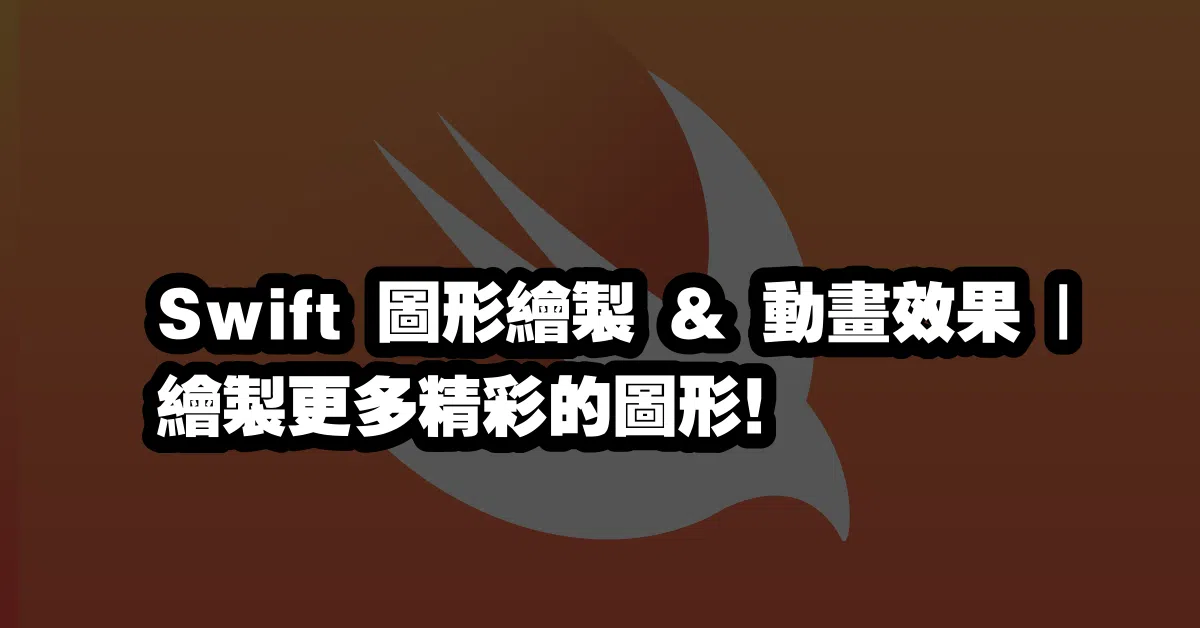# Swift 圖形繪製 & 動畫效果 | 繪製更多精彩的圖形！🎨

Swift 是一種非常強大的程式語言，它可以讓開發者繪製出更多精彩的圖形和動畫效果！在本文中，我們將介紹如何使用 Swift 來繪製圖形和動畫效果，以及如何使用 UIKitCore Graphics 來繪製出更多精彩的圖形！

``````import UIKit
import CoreGraphics
``````

``````let path = UIBezierPath()
path.move(to: CGPoint(x: 0, y: 0))
path.close()

let shapeLayer = CAShapeLayer()
shapeLayer.path = path.cgPath

``````

``````let context = UIGraphicsGetCurrentContext()

// 繪製圓形
context?.addEllipse(in: CGRect(x: 0, y: 0, width: 100, height: 100))
context?.setFillColor(UIColor.red.cgColor)
context?.fillPath()

// 繪製線條
context?.move(to: CGPoint(x: 0, y: 0))
context?.setStrokeColor(UIColor.blue.cgColor)
context?.setLineWidth(2.0)
context?.strokePath()

// 繪製文字
let text = "Hello World!"
let font = UIFont.systemFont(ofSize: 16.0)
let textAttributes = [NSAttributedString.Key.font: font]
let textSize = text.size(withAttributes: textAttributes)
let textRect = CGRect(x: 0, y: 0, width: textSize.width, height: textSize.height)
text.draw(in: textRect, withAttributes: textAttributes)
``````

## 延伸閱讀本站文章